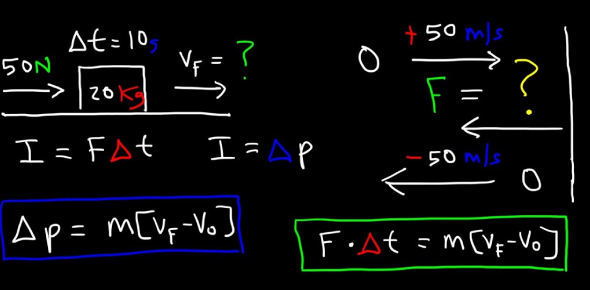# Momentum And Impulse

13 Questions | Attempts: 1275
ShareSettings• 1.
The diagram below shows a 4.0-kilogram cart moving to the right and a 6.0-kilogram cart moving to the left on a horizontal frictionless surface. When the two carts collide they lock together. The magnitude of the total momentum of the two-cart system after the collision is
• A.

0.0 Ns

• B.

15 Ns

• C.

6.0 Ns

• D.

30.0 Ns

• 2.
A 0.050-kilogram bullet is fired from a 4.0 kilogram rifle that is initially at rest. If the bullet leaves the rifle with momentum having a magnitude of 20.0 Ns, the rifle will recoil with a momentum having a magnitude of
• A.

1,600 Ns

• B.

20.0 Ns

• C.

80.0 Ns

• D.

0.25 Ns

• 3.
What impulse must be applied to a 25.0 kg cart to cause a velocity change of 12.0 m/s?
• A.

0.48 Ns

• B.

2.1 Ns

• C.

13.0 Ns

• D.

300.0 Ns

• 4.
A 6.0 × 10-3 kg insect is flying horizontally with a momentum of 4.8 × 10-2 Ns headwind. What is its resulting velocity relative to the ground?
• A.

8.0 m/s

• B.

6.0 m/s

• C.

0.80 m/s

• D.

0.60 m/s

• 5.
A ball is hit with a bat. A student determines that the momentum of the ball is 1.0 Ns.  What is the mass of the ball if it has a velocity of 2.0 meters per second?
• A.

0 kg

• B.

0.50 kg

• C.

1.0 kg

• D.

2.0 kg

• 6.
A 0.40-kg flashlight is constructed to withstand a specific force of impact that protects the bulb. A standard 0.10 second is used as time-of-impact in calculating a flashlight’s specifications.  What is the impact force on the 0.40 kg flashlight if it falls 2.5 meters and hits a concrete floor at 7.0 m/s, stopping in 0.10 seconds?
• A.

1.0 N

• B.

2.8 N

• C.

28 N

• D.

98 N

• 7.
A 60.0 kg student on ice skates stands at rest on a frictionless frozen pond holding a 10.-kg brick. He throws the brick east with a speed of 18 m/s.  What is the resulting velocity of the student?
• A.

3.0 m/s west

• B.

3.0 m/s east

• C.

18 m/s west

• D.

18 m/s east

• 8.
A train car with a mass of 3.0 E 4 kg traveling north at 1.5 m/s collides and couples with a 3.2 E 4 kg train car going south at 0.80 m/s. What is the velocity of the coupled cars after the collision?
• A.

0.31 m/s north

• B.

0.31 m/s south

• C.

0.97 m/s north

• D.

0.97 m/s south

• 9.
A 1,000-kg cannon fires a 10 kg projectile horizontally at a velocity of 300 m/s. What is the recoil velocity of the cannon?
• A.

0.3 m/s

• B.

3 m/s

• C.

30 m/s

• D.

300 m/s

• 10.
A student on roller skates throws a basketball forward. How does the momentum of the student on skates compare to the momentum of the basketball?
• A.

The velocity of the student is equal in magnitude but opposite in direction to the velocity of the basketball.

• B.

The velocity of the student is equal in magnitude and in the same direction as the velocity of the basketball.

• C.

The product of the mass times the velocity of the student is equal in magnitude and in the same direction as the product of the mass times the velocity of the basketball.

• D.

The product of the mass times the velocity of the student is equal in magnitude but opposite in direction to the product of the mass times the velocity of the basketball.

• 11.
During batting practice, a 0.30 kg baseball is hit with a bat that exerts a force of 350 N on the ball. The ball left the bat at 80. 0 m/s. If the incoming pitch was traveling at 60.0 m/s, how long did the ball stay in contact with the bat?
• A.

0.12 s

• B.

0.50 s

• C.

0.85 s

• D.

1.4 s

• 12.
Which is an acceptable unit for impulse?
• A.

N•m

• B.

J•s

• C.

J/s

• D.

kg•m/s

• 13.
A 3.0 kilogram steel block is at rest on a frictionless horizontal surface. A 1.0 kilogram lump of clay is propelled horizontally at 6.0 meters per second toward the block as shown in the diagram below.   Upon collision, the clay and steel block stick together and move to the right with a speed of
• A.

1.5 m/s

• B.

3.0 m/s

• C.

2.0 m/s

• D.

6.0 m/s

## Related TopicsBack to top
×

Wait!
Here's an interesting quiz for you.